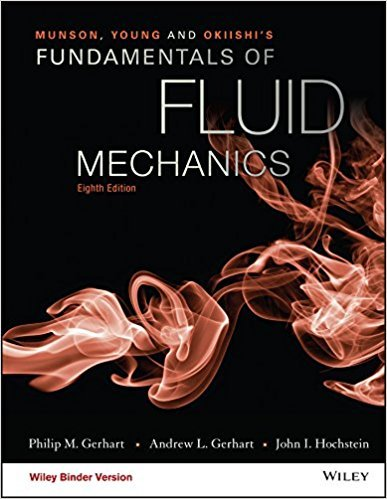×
Get Full Access to Fundamentals Of Fluid Mechanics - 8 Edition - Chapter 11.1 - Problem 11.3
Get Full Access to Fundamentals Of Fluid Mechanics - 8 Edition - Chapter 11.1 - Problem 11.3

×

# Consider the flow process in Fig. P11.3. Does the fluid flowfrom left to right or fromISBN: 9781119080701 456

## Solution for problem 11.3 Chapter 11.1

Fundamentals of Fluid Mechanics | 8th Edition

• Textbook Solutions
• 2901 Step-by-step solutions solved by professors and subject experts
• Get 24/7 help from StudySoup virtual teaching assistantsFundamentals of Fluid Mechanics | 8th Edition

4 5 1 275 Reviews
19
5
Problem 11.3

Consider the flow process in Fig. P11.3. Does the fluid flowfrom left to right or from right to left? Justify your answer. Assumeas ideal gas with constant specific heats.

Step-by-Step Solution:
Step 1 of 3

I s ::. -1 t= Hl- l'\~e ~o fru(,-t w;t.V,- y_ > J ( ..> d~ 0 v ::. t"/u\]:_M~ ~ h') ~ l- ,.> - c\~:....a~ we '-'ve - u ~6\ "t,r -H;:v:·V - O\J t\o~ {:),,;'> -o v:. :ies..JS ) .\;I...

Step 2 of 3

Step 3 of 3

##### ISBN: 9781119080701

This textbook survival guide was created for the textbook: Fundamentals of Fluid Mechanics, edition: 8. The answer to “Consider the flow process in Fig. P11.3. Does the fluid flowfrom left to right or from right to left? Justify your answer. Assumeas ideal gas with constant specific heats.” is broken down into a number of easy to follow steps, and 29 words. This full solution covers the following key subjects: . This expansive textbook survival guide covers 112 chapters, and 1357 solutions. Since the solution to 11.3 from 11.1 chapter was answered, more than 210 students have viewed the full step-by-step answer. Fundamentals of Fluid Mechanics was written by and is associated to the ISBN: 9781119080701. The full step-by-step solution to problem: 11.3 from chapter: 11.1 was answered by , our top Science solution expert on 03/16/18, 03:21PM.

Unlock Textbook Solution